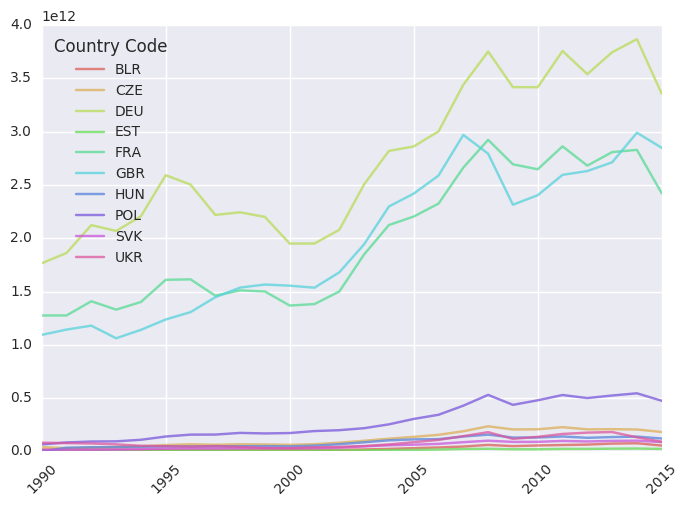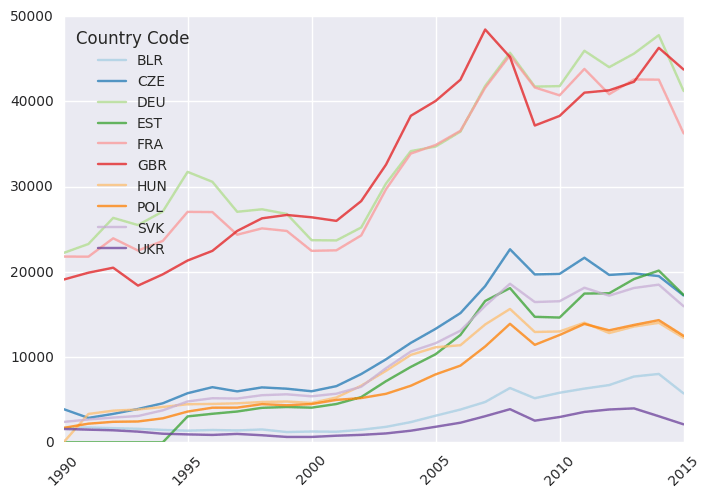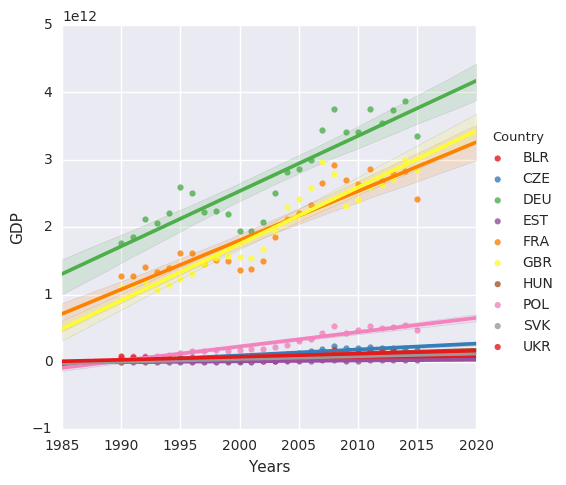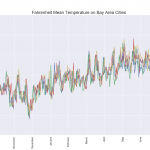## The World Bank GDP Analysis using Pandas and Seaborn Python libraries

Pandas and Seaborn are one of the most useful data science related Python libraries. The first one provides an easy to use and high-performance data structures and methods for data manipulation. The latter is build on top of matplotlib and provides a high-level interface for drawing attractive statistical graphics. How do they work?

Let’s check it out using World Bank GDP data from 10 central European countries – Poland, Germany, Belarus, the Czech Republic, the Slovak Republic, Hungary, Estonia, France, Ukraine and the United Kingdom.

## What are we looking for?

The question – How far in economic development eastern Europe countries are relative to developed countries like Germany and France?

To answer it we need to analyze four GDP factors – GDP per capita (US\$), GDO per capita growth (annual %), GDP growth (annual %) and GDP (current US\$).

The data from the World Bank (from the World Development Indicators website to be exact) are in an open format and have good history records for many countries that include a number of economic and social indicators.

We chose the years 1990 – 2016 because only these were available for the selected indicators.

You can find the data here.

## The code

First, we load the data from the CSV file. Then we remove the last 5 lines, because they contain empty values and information about the date of the last data update. In addition, we have to remove the column with the year 2016, because, as it turned out it is empty (no data). “gdp.replace” is responsible for the replacement of two dots, symbolizing the empty NaN.

```import numpy as np
import pandas as pd
import matplotlib as mpl
import matplotlib.pyplot as plt

#we take only data, not additional informations
gdp = gdp[0:-5]
#delete empty column
del gdp['2016 [YR2016]']
#replace '..' string with nan values
gdp.replace('..', np.nan, inplace=True)```

In the course of further work with DataFrame I received mysterious errors and at first, I was not able to determine what was wrong. After some time I decided to check the types of the individual columns:

```gdp.dtypes

Country Name      object
Country Code      object
Series Name       object
Series Code       object
1990 [YR1990]     object
1991 [YR1991]     object
1992 [YR1992]     object
1993 [YR1993]     object
1994 [YR1994]     object
1995 [YR1995]     object
1996 [YR1996]    float64
1997 [YR1997]    float64
1998 [YR1998]    float64
1999 [YR1999]    float64
2000 [YR2000]    float64
2001 [YR2001]    float64
2002 [YR2002]    float64
2003 [YR2003]    float64
2004 [YR2004]    float64
2005 [YR2005]    float64
2006 [YR2006]    float64
2007 [YR2007]    float64
2008 [YR2008]    float64
2009 [YR2009]    float64
2010 [YR2010]    float64
2011 [YR2011]    float64
2012 [YR2012]    float64
2013 [YR2013]    float64
2014 [YR2014]    float64
2015 [YR2015]    float64
dtype: objec```

To my surprise dates from 1990 to 1995 didn’t have the data type float64 only object, so I decided to be sure all the columns of years to convert to numeric values. For this purpose, I select  columns from 4 up to the end (that is, all of the years) and with use of “apply” method ‘I applied the function “pd.to_numeric“. It converts all years to a floating point number.

```# some of the colums are objects, we have to convert to floats,
#then pivot_table will take them into consideration
col_list = gdp.columns[4:].values
gdp[col_list]=gdp[col_list].apply(pd.to_numeric)```

In each row, was the name of the country, its code, the name of a series of data from the World Bank, its code, and in subsequent columns the years. Such arrangement of the data was not too comfortable so I decided to reindex the table using the functions “pivot_table

```#reindex all table, create pivot view
pv2 = pd.pivot_table(gdp,index=['Series Name','Country Code'], dropna=False, fill_value=0.0)
# set the years
pv2.columns= np.arange(1990,2016)```

This has changed dataframe from form:To this one.That way I can pull any economic indicator and immediately have all the countries along with all the years.

Now I can easily visualize 4 selected indicators. For nicer graphs import Seaborn and set the color palette so that each line on the graph was plotted with a different color. Try comparing charts with and without Seaborn.

Drawing directly with the pandas is really simple – just for our pivot table choose the interesting indicator, then transpose the data (function .T) and plot (, plot ‘).

```import seaborn as sns
palette = sns.color_palette("Paired", 10)
sns.set_palette(palette)

pv2.loc['GDP (current US\$)'].T.plot(alpha=0.75, rot=45)
pv2.loc['GDP per capita (current US\$)'].T.plot(alpha-0.8, rot=45)
pv2.loc['GDP per capita (current US\$)'].T.plot(alpha=0.75, rot=45)
pv2.loc['GDP growth (annual %)'].T.plot(alpha=0.75, rot=45)```

The first two charts

1. GDP (current US\$), data from World bank2. GDP per capita, data from World bankLet’s try to perform a simple regression from the GDP data to see if there is a chance that one day we can catch up with Germany. This time we will use the “lmplot” function from the Seaborn library, except that the data must lead to a form of time series.

From the data in the form of a table with countries as columns, we need to create a table in which we will have only three columns [years, the country GDP]. We do this through a series of operations, the removal of the index, because our table at the beginning of the year is indexed (unique rows), changes of the name of the column. The key operation here is the “melt” function that transmits the data from the column and adds them into the next rows. So that we are able to make the following transformation. The attached images omitted part of the columns and rows but I hope its clear.```#seaborn plots
plot_data = pv2.loc['GDP (current US\$)'].T.reset_index()
plot_data.rename(columns={'index':'Years'}, inplace=True)
# unpivot the data, change from table view, where we have columns for each
# country, to big long time series data, [year, country code, value]
melt_data = pd.melt(plot_data, id_vars=['Years'],var_name='Country')
melt_data.rename(columns={'value':'GDP'}, inplace=True)
sns.lmplot(x="Years", y="GDP", hue="Country", data=melt_data, palette="Set1");```

We should get a result similar to this: